×
Get Full Access to Chemistry - 11 Edition - Chapter 3 - Problem 121p
Get Full Access to Chemistry - 11 Edition - Chapter 3 - Problem 121p

×

# Solved: Calculate the number of cations and anions in each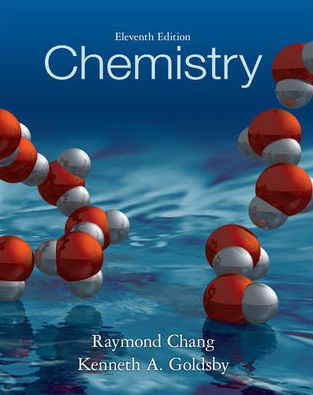ISBN: 9780073402680 118

## Solution for problem 121P Chapter 3

Chemistry | 11th Edition

• Textbook Solutions
• 2901 Step-by-step solutions solved by professors and subject experts
• Get 24/7 help from StudySoup virtual teaching assistantsChemistry | 11th Edition

4 5 1 401 Reviews
10
2
Problem 121P

Calculate the number of cations and anions in each of the following compounds: (a) 0.764 g of CsI, (b) $$72.8\ \text{g}\ K_2Cr_2O_7$$, (c) $$6.54\ \text{g}\ \text{of}\ Hg_2(NO_3)_2$$.

Step-by-Step Solution:

Step 1 of 3

Here, we are asked to calculate the number of cations and anions in the given compounds

(a) Given: 0.764 g of CsI

First, let’s find the number of moles of CsI: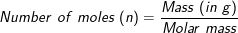Molar mass of CsI (Caesium iodide) = 259.81 g/mol

We know 1 mole of any unit contains Avogadro’s number of particles. Here, 1 mole of the CsI element contains Avogadro’s number of ions,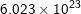.

Therefore, number of ions will be:

=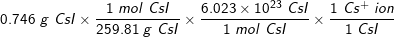=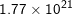ions

Cs (Caesium) is a metal and hence these ions are the cations. Hence, there are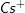ions.

The compound, CsI contains 1 atom of Cs and 1 atom of I (Iodine). A compound always contains an equal number of cations and anions in the neutral state. Hence, CsI hasanions, i.e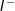ions.

Step 2 of 3

Step 3 of 3

##### ISBN: 9780073402680

This full solution covers the following key subjects: anions, calculate, cations, compounds, CSI. This expansive textbook survival guide covers 25 chapters, and 3591 solutions. The answer to “?Calculate the number of cations and anions in each of the following compounds: (a) 0.764 g of CsI, (b) $$72.8\ \text{g}\ K_2Cr_2O_7$$, (c) $$6.54\ \text{g}\ \text{of}\ Hg_2(NO_3)_2$$.” is broken down into a number of easy to follow steps, and 27 words. This textbook survival guide was created for the textbook: Chemistry, edition: 11. Chemistry was written by and is associated to the ISBN: 9780073402680. The full step-by-step solution to problem: 121P from chapter: 3 was answered by , our top Chemistry solution expert on 11/08/17, 03:59AM. Since the solution to 121P from 3 chapter was answered, more than 10129 students have viewed the full step-by-step answer.

Unlock Textbook Solution